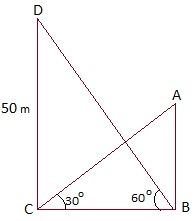Guru

# The angle of elevation of the top of a building from the foot of the tower is 30° and the angle of elevation of the top of the tower from the foot of the building is 60°. If the tower is 50 m high, find the height of the building. Q.9

• 0

What is the best way to solve the problem of question no.9 of class 10th of applications of trigonometry of exercise 9.1 of math, what is the best to solve this problem sir give me the best way to solve this problem The angle of elevation of the top of a building from the foot of the tower is 30° and the angle of elevation of the top of the tower from the foot of the building is 60°. If the tower is 50 m high, find the height of the building.

Share

1. Let CD be the height of the tower. AB be the height of the building. BC be the distance between the foot of the building and the tower. Elevation is 30 degree and 60 degree from the tower and the building respectively.In right ΔBCD,

tan 60° = CD/BC

√3 = 50/BC

BC = 50/√3 …(1)

Again,

In right ΔABC,

tan 30° = AB/BC

⇒ 1/√3 = AB/BC

Use result obtained in equation (1)

AB = 50/3

Thus, the height of the building is 50/3 m.

• 0